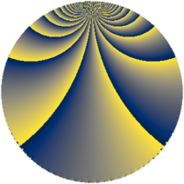# Properties

 Label 1368.3.ciLevel $1368$ Weight $3$ Character orbit 1368.ci Rep. character $\chi_{1368}(425,\cdot)$ Character field $\Q(\zeta_{6})$ Dimension $240$ Sturm bound $720$

# Related objects

## Defining parameters

 Level: $$N$$ $$=$$ $$1368 = 2^{3} \cdot 3^{2} \cdot 19$$ Weight: $$k$$ $$=$$ $$3$$ Character orbit: $$[\chi]$$ $$=$$ 1368.ci (of order $$6$$ and degree $$2$$) Character conductor: $$\operatorname{cond}(\chi)$$ $$=$$ $$171$$ Character field: $$\Q(\zeta_{6})$$ Sturm bound: $$720$$

## Dimensions

The following table gives the dimensions of various subspaces of $$M_{3}(1368, [\chi])$$.

Total New Old
Modular forms 976 240 736
Cusp forms 944 240 704
Eisenstein series 32 0 32

## Trace form

 $$240q - 4q^{3} + 12q^{9} + O(q^{10})$$ $$240q - 4q^{3} + 12q^{9} - 18q^{11} + 8q^{15} + 108q^{17} - 6q^{19} + 600q^{25} - 40q^{27} - 24q^{31} - 72q^{33} - 52q^{39} + 54q^{41} - 216q^{43} + 160q^{45} - 108q^{47} - 840q^{49} - 14q^{51} - 4q^{57} - 126q^{59} + 20q^{63} + 432q^{65} - 84q^{67} + 16q^{69} - 18q^{73} - 214q^{75} - 288q^{77} - 92q^{81} - 124q^{87} + 216q^{89} - 96q^{91} + 144q^{93} - 468q^{95} - 180q^{97} + 170q^{99} + O(q^{100})$$

## Decomposition of $$S_{3}^{\mathrm{new}}(1368, [\chi])$$ into newform subspaces

The newforms in this space have not yet been added to the LMFDB.

## Decomposition of $$S_{3}^{\mathrm{old}}(1368, [\chi])$$ into lower level spaces

$$S_{3}^{\mathrm{old}}(1368, [\chi]) \cong$$ $$S_{3}^{\mathrm{new}}(171, [\chi])$$$$^{\oplus 4}$$$$\oplus$$$$S_{3}^{\mathrm{new}}(342, [\chi])$$$$^{\oplus 3}$$$$\oplus$$$$S_{3}^{\mathrm{new}}(684, [\chi])$$$$^{\oplus 2}$$